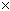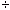Question from Toi: How much would it cost if I had an item that was 54x14x8 and it was \$28.00 per cubic foot? Hi Toi. By multiplying the three length dimensions together, you get a volume. If you multiply volume by (cost per unit volume), you get cost. You didn't specify, but if your length measurements above are in feet, then you just calculate 5414828. If your measurements are in inches, you need to divide this first calculation by twelve (inches per foot) for each time you use inches (three). That means 5414828121212. Hope this helps, Stephen La Rocque. >